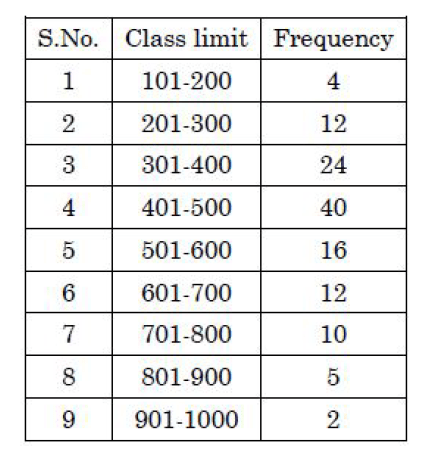# GEOGRAPHICAL TECHNIQUES PYQS JUNE & DEC 2020 NTA UGC NET

GEOGRAPHICAL TECHNIQUES (CARTOGRAPHY & STATS) PYQS JUNE & DEC 2020 NTA UGC NET

GEOGRAPHICAL TECHNIQUES PYQS JUNE 2020 NTA UGC NET, GEOGRAPHICAL TECHNIQUES  NTA UGC NET DEC 2020,GEOGRAPHICAL TECHNIQUES  PYQS UGC NET JUNE 2020, GEOGRAPHICAL TECHNIQUES  PYQS DEC 2020 NTA UGC NET PYQS GEOGRAPHY, GEOGRAPHICAL TECHNIQUES  PYQS UGC NET, PRACTICAL GEOGRAPHY PYQS NTA UGC NET, CARTOGRAPHY PYQS NTAUGC NET, STATS PYQS NTAUGC NET, GEOGRAPHY PYQS UGC NET,

(PDF) For download PDF file of 2020 UGC NET – go to the bottom of this post.

Q.1. Read the following statement regarding Hypsometric curve (NTA UGC NET JUNE 2020)

(A) It shows the area – height relationship of a drainage basin.

(B) A convex curve indicates that bulk of the basin’s area remains at relatively low elevation.

(C) A concave curve in characterized by diffusive hill slope processes such as land sliding, soil creep etc.

(D) The simple way to characterize the shape of hypsometric curve for any drainage basin is to calculate hypsometric integral (HD)

Choose the correct answer from the options given below:

(1) (A), (B) and (C) are correct

(2) (B) and (C) are correct

(3) (A) and (D) are correct

(4) (A), (B), (C) and (D) are correct

Q.2. Two dimensional diagrams are also known as (NTA UGC NET JUNE 2020)

(1) Size

(2) Volume

(3) Ratio

(4) Area

Q.3. In which of the following maps average slope and relative relief are shown simultaneously (NTA UGC NET JUNE 2020)

(1) Trachographic map

(2) Planimetric map

(3) Hypsometric map

(4) Morphological map

Q.4. Histogram is associated with which one of the following data distribution (NTA UGC NET JUNE 2020)

(1) Discrete frequency distribution

(2) Continious frequency distribution

(3) Log-normal frequency distribution

(4) Un-classified data

Q.5. Which one of the following curves is correct to show the cumulative frequency distribution? (NTA UGC NET JUNE 2020)

(1) Frequency curve

(2) Ogive

(3) Log-normal eurve

(4) Semi-log curve

Q.6. Which one of the following techniques is correct to show inequality of two given distribution (NTA UGC NET JUNE 2020)

(1) Rn statistics

(2) X2 test

(3) Correlation Coefficient

(4) Lorenz curve

Q.7. Match List I with List II: (NTA UGC NET JUNE 2020)

List I – (Settlement)List II – (Pattern)

(I) Uniform

(II) Random

(III) More clustered than uniform

(IV) Clustered

Choose the correct answer from the options given below:

 A B C D (1) II I IV III (2) I II IV III (3) II I III IV (4) II IV I III

Q.8. Which one of the following percentage of frequencies between the range of 0 to + 1.00 (σ) standard deviation (NTA UGC NET JUNE 2020)(1) 34.13%

(2) 38.23%

(3) 46.20%

(4) 47.73%

Comprehension:

PassageConsider the following data and answer questions: 9 – 13

Q.9. Which one of the following is the cumulative frequency for the class limit 401 – 500 from the given data set. (NTA UGC NET JUNE 2020)

(1) 24

(2) 40

(3) 16

(4) 80

Q.10. Which one of the following is the cumulative frequency of the entire data set (NTA UGC NET JUNE 2020)

(1) 125

(2) 24

(3) 40

(4) 118

Q.11. Which one of the following is the relative frequency in percentage for class limit 301- 400 from the given data set. (NTA UGC NET JUNE 2020)

(1) 18.2

(2) 19.2

(3) 20.2

(4) 24.2

Q.12. Which one of the following is the mode value for the given data set (NTA UGC NET JUNE 2020)

(1) 483

(2) 125

(3) 400.5

(4) 441

Q.13. Which one of the following is the arithmetic mean value for the given data set (NTA UGC NET JUNE 2020)

(1) 125

(2) 40

(3) 483

(4) 603